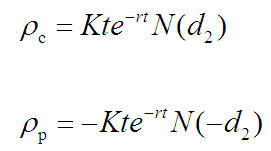# Option Greeks: Rho

Rho is a measure of an option's sensitivity to changes in the risk free interest rate.

It is expressed as the amount of money an option will lose or gain with a 1% change in interest rates.

For example, consider a call option with a rho of 0.05. This means that if the interest rates increase by 1%, the price of the option will increase by \$0.05.

Calculating Rho

Rho is calculated using the following formula:Rho is the least used and least important greek.

Long calls and short puts have positive rho, that is, the option price will increase with an increase in interest rates and it will decrease with a decrease in interest rates.

Short calls and long puts have negative rho, that is, the option price will increase with a decrease in interest rates and it will decrease with a increase in interest rates.

For both call and put options, the more the time remaining for expiry, the higher is the impact of interest rates. Unless the option has very long life, the changes in interest rates affect the premium only modestly.

Deep out-of-the-money options have low rho compared to at-the-money and deep in-the-money options.